Anzeige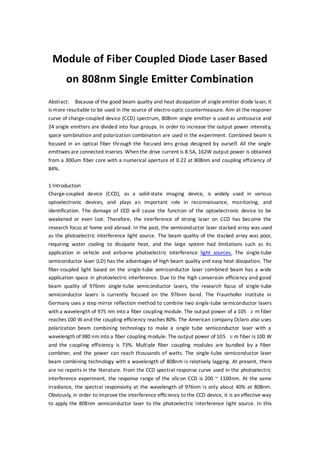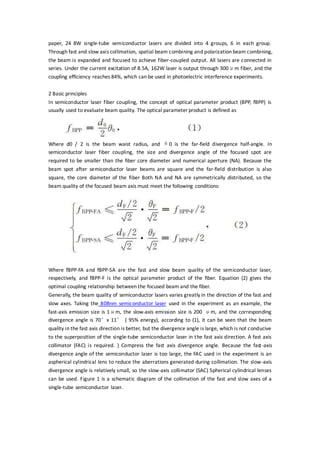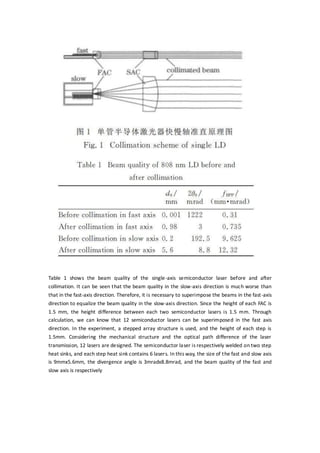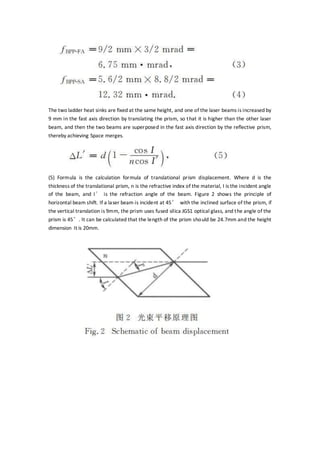Anzeige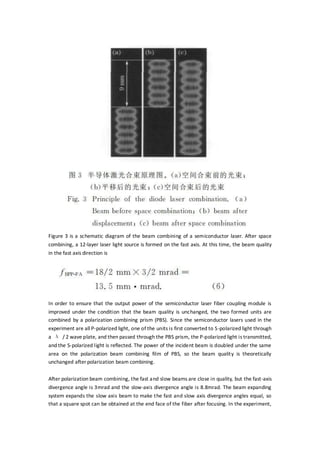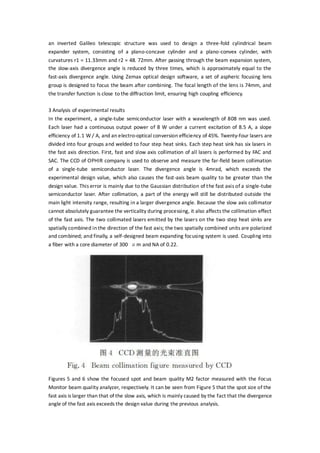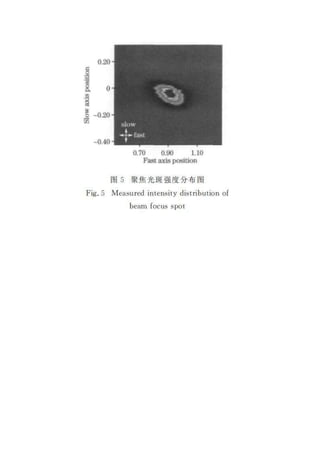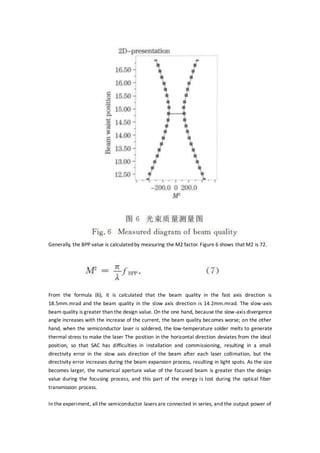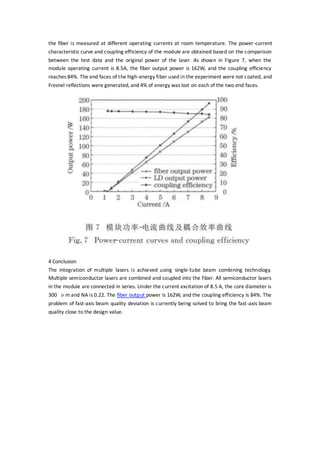Nächste SlideShareLecture 10 focusing and colimating
1 von 9
Anzeige

### Module of fiber coupled diode laser based on 808nm single emitter combination

1. Module of Fiber Coupled Diode Laser Based on 808nm Single Emitter Combination Abstract: Because of the good beam quality and heat dissipation of single emitter diode laser, it is more resuitable to be used in the source of electro-optic countermeasure. Aim at the responer curve of charge-coupled device (CCD) spectrum, 808nm single emitter is used as unitsource and 24 single emitters are divided into four groups. In order to increase the output power intensity, space sombination and polarization combination are used in the experiment. Combined beam is focused in an optical fiber through the focused lens group designed by ourself. All the single emittwes are connected inseries. When the drive current is 8.5A, 162W output power is obtained from a 300um fiber core with a numerical aperture of 0.22 at 808nm and coupling efficiency of 84%. 1 Introduction Charge-coupled device (CCD), as a solid-state imaging device, is widely used in various optoelectronic devices, and plays an important role in reconnaissance, monitoring, and identification. The damage of CCD will cause the function of the optoelectronic device to be weakened or even lost. Therefore, the interference of strong laser on CCD has become the research focus at home and abroad. In the past, the semiconductor laser stacked array was used as the photoelectric interference light source. The beam quality of the stacked array was poor, requiring water cooling to dissipate heat, and the large system had limitations such as its application in vehicle and airborne photoelectric interference light sources. The single-tube semiconductor laser (LD) has the advantages of high beam quality and easy heat dissipation. The fiber-coupled light based on the single-tube semiconductor laser combined beam has a wide application space in photoelectric interference. Due to the high conversion efficiency and good beam quality of 976nm single-tube semiconductor lasers, the research focus of single-tube semiconductor lasers is currently focused on the 976nm band. The Fraunhofer Institute in Germany uses a step mirror reflection method to combine two single-tube semiconductor lasers with a wavelength of 975 nm into a fiber coupling module. The output power of a 105 μm fiber reaches 100 W and the coupling efficiency reaches 80%. The American company Oclaro also uses polarization beam combining technology to make a single tube semiconductor laser with a wavelength of 980 nm into a fiber coupling module. The output power of 105 μm fiber is 100 W and the coupling efficiency is 73%. Multiple fiber coupling modules are bundled by a fiber combiner, and the power can reach thousands of watts. The single-tube semiconductor laser beam combining technology with a wavelength of 808nm is relatively lagging. At present, there are no reports in the literature. From the CCD spectral response curve used in the photoelectric interference experiment, the response range of the silicon CCD is 200 ~ 1100nm. At the same irradiance, the spectral responsivity at the wavelength of 976nm is only about 40% at 808nm. Obviously, in order to improve the interference efficiency to the CCD device, it is an effective way to apply the 808nm semiconductor laser to the photoelectric interference light source. In this
2. paper, 24 8W single-tube semiconductor lasers are divided into 4 groups, 6 in each group. Through fast and slow axis collimation, spatial beam combining and polarization beam combining, the beam is expanded and focused to achieve fiber-coupled output. All lasers are connected in series. Under the current excitation of 8.5A, 162W laser is output through 300μm fiber, and the coupling efficiency reaches 84%, which can be used in photoelectric interference experiments. 2 Basic principles In semiconductor laser fiber coupling, the concept of optical parameter product (BPP, fBPP) is usually used to evaluate beam quality. The optical parameter product is defined as Where d0 / 2 is the beam waist radius, and θ0 is the far-field divergence half-angle. In semiconductor laser fiber coupling, the size and divergence angle of the focused spot are required to be smaller than the fiber core diameter and numerical aperture (NA). Because the beam spot after semiconductor laser beams are square and the far-field distribution is also square, the core diameter of the fiber Both NA and NA are symmetrically distributed, so the beam quality of the focused beam axis must meet the following conditions: Where fBPP-FA and fBPP-SA are the fast and slow beam quality of the semiconductor laser, respectively, and fBPP-F is the optical parameter product of the fiber. Equation (2) gives the optimal coupling relationship between the focused beam and the fiber. Generally, the beam quality of semiconductor lasers varies greatly in the direction of the fast and slow axes. Taking the 808nm semiconductor laser used in the experiment as an example, the fast-axis emission size is 1μm, the slow-axis emission size is 200 μm, and the corresponding divergence angle is 70°x 11° ( 95% energy), according to (1), it can be seen that the beam quality in the fast axis direction is better, but the divergence angle is large, which is not conducive to the superposition of the single-tube semiconductor laser in the fast axis direction. A fast axis collimator (FAC) is required. ) Compress the fast axis divergence angle. Because the fast-axis divergence angle of the semiconductor laser is too large, the FAC used in the experiment is an aspherical cylindrical lens to reduce the aberrations generated during collimation. The slow-axis divergence angle is relatively small, so the slow-axis collimator (SAC) Spherical cylindrical lenses can be used. Figure 1 is a schematic diagram of the collimation of the fast and slow axes of a single-tube semiconductor laser.
3. Table 1 shows the beam quality of the single-axis semiconductor laser before and after collimation. It can be seen that the beam quality in the slow-axis direction is much worse than that in the fast-axis direction. Therefore, it is necessary to superimpose the beams in the fast-axis direction to equalize the beam quality in the slow-axis direction. Since the height of each FAC is 1.5 mm, the height difference between each two semiconductor lasers is 1.5 mm. Through calculation, we can know that 12 semiconductor lasers can be superimposed in the fast axis direction. In the experiment, a stepped array structure is used, and the height of each step is 1.5mm. Considering the mechanical structure and the optical path difference of the laser transmission, 12 lasers are designed. The semiconductor laser is respectively welded on two step heat sinks, and each step heat sink contains 6 lasers. In this way, the size of the fast and slow axis is 9mmx5.6mm, the divergence angle is 3mradx8.8mrad, and the beam quality of the fast and slow axis is respectively
4. The two ladder heat sinks are fixed at the same height, and one of the laser beams is increased by 9 mm in the fast axis direction by translating the prism, so that it is higher than the other laser beam, and then the two beams are superposed in the fast axis direction by the reflective prism, thereby achieving Space merges. (5) Formula is the calculation formula of translational prism displacement. Where d is the thickness of the translational prism, n is the refractive index of the material, I is the incident angle of the beam, and I′ is the refraction angle of the beam. Figure 2 shows the principle of horizontal beam shift. If a laser beam is incident at 45° with the inclined surface of the prism, if the vertical translation is 9mm, the prism uses fused silica JGS1 optical glass, and the angle of the prism is 45°. It can be calculated that the length of the prism should be 24.7mm and the height dimension It is 20mm.
5. Figure 3 is a schematic diagram of the beam combining of a semiconductor laser. After space combining, a 12-layer laser light source is formed on the fast axis. At this time, the beam quality in the fast axis direction is In order to ensure that the output power of the semiconductor laser fiber coupling module is improved under the condition that the beam quality is unchanged, the two formed units are combined by a polarization combining prism (PBS). Since the semiconductor lasers used in the experiment are all P-polarized light, one of the units is first converted to S-polarized light through a λ / 2 wave plate, and then passed through the PBS prism, the P-polarized light is transmitted, and the S-polarized light is reflected. The power of the incident beam is doubled under the same area on the polarization beam combining film of PBS, so the beam quality is theoretically unchanged after polarization beam combining. After polarization beam combining, the fast and slow beams are close in quality, but the fast-axis divergence angle is 3mrad and the slow-axis divergence angle is 8.8mrad. The beam expanding system expands the slow axis beam to make the fast and slow axis divergence angles equal, so that a square spot can be obtained at the end face of the fiber after focusing. In the experiment,
6. an inverted Galileo telescopic structure was used to design a three-fold cylindrical beam expander system, consisting of a plano-concave cylinder and a plano-convex cylinder, with curvatures r1 = 11.33mm and r2 = 48. 72mm. After passing through the beam expansion system, the slow-axis divergence angle is reduced by three times, which is approximately equal to the fast-axis divergence angle. Using Zemax optical design software, a set of aspheric focusing lens group is designed to focus the beam after combining. The focal length of the lens is 74mm, and the transfer function is close to the diffraction limit, ensuring high coupling efficiency. 3 Analysis of experimental results In the experiment, a single-tube semiconductor laser with a wavelength of 808 nm was used. Each laser had a continuous output power of 8 W under a current excitation of 8.5 A, a slope efficiency of 1.1 W / A, and an electro-optical conversion efficiency of 45%. Twenty-four lasers are divided into four groups and welded to four step heat sinks. Each step heat sink has six lasers in the fast axis direction. First, fast and slow axis collimation of all lasers is performed by FAC and SAC. The CCD of OPHIR company is used to observe and measure the far-field beam collimation of a single-tube semiconductor laser. The divergence angle is 4mrad, which exceeds the experimental design value, which also causes the fast-axis beam quality to be greater than the design value. This error is mainly due to the Gaussian distribution of the fast axis of a single-tube semiconductor laser. After collimation, a part of the energy will still be distributed outside the main light intensity range, resulting in a larger divergence angle. Because the slow axis collimator cannot absolutely guarantee the verticality during processing, it also affects the collimation effect of the fast axis. The two collimated lasers emitted by the lasers on the two step heat sinks are spatially combined in the direction of the fast axis; the two spatially combined units are polarized and combined; and finally, a self-designed beam expanding focusing system is used. Coupling into a fiber with a core diameter of 300 μm and NA of 0.22. Figures 5 and 6 show the focused spot and beam quality M2 factor measured with the Focus Monitor beam quality analyzer, respectively. It can be seen from Figure 5 that the spot size of the fast axis is larger than that of the slow axis, which is mainly caused by the fact that the divergence angle of the fast axis exceeds the design value during the previous analysis.
7. Generally, the BPP value is calculatedby measuring the M2 factor. Figure 6 shows that M2 is 72. From the formula (6), it is calculated that the beam quality in the fast axis direction is 18.5mm.mrad and the beam quality in the slow axis direction is 14.2mm.mrad. The slow-axis beam quality is greater than the design value. On the one hand, because the slow-axis divergence angle increases with the increase of the current, the beam quality becomes worse; on the other hand, when the semiconductor laser is soldered, the low-temperature solder melts to generate thermal stress to make the laser The position in the horizontal direction deviates from the ideal position, so that SAC has difficulties in installation and commissioning, resulting in a small directivity error in the slow axis direction of the beam after each laser collimation, but the directivity error increases during the beam expansion process, resulting in light spots. As the size becomes larger, the numerical aperture value of the focused beam is greater than the design value during the focusing process, and this part of the energy is lost during the optical fiber transmission process. In the experiment, all the semiconductor lasers are connected in series, and the output power of
8. the fiber is measured at different operating currents at room temperature. The power-current characteristic curve and coupling efficiency of the module are obtained based on the comparison between the test data and the original power of the laser. As shown in Figure 7, when the module operating current is 8.5A, the fiber output power is 162W, and the coupling efficiency reaches 84%. The end faces of the high-energy fiber used in the experiment were not coated, and Fresnel reflections were generated, and 4% of energy was lost on each of the two end faces. 4 Conclusion The integration of multiple lasers is achieved using single-tube beam combining technology. Multiple semiconductor lasers are combined and coupled into the fiber. All semiconductor lasers in the module are connected in series. Under the current excitation of 8.5 A, the core diameter is 300 μm and NA is 0.22. The fiber output power is 162W, and the coupling efficiency is 84%. The problem of fast-axis beam quality deviation is currently being solved to bring the fast-axis beam quality close to the design value.
Anzeige